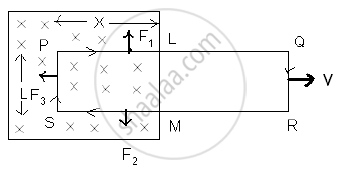# State Lenz'S Law. - Physics

State Lenz's Law.

#### Solution 1

Lenz’s law: It is stated that the direction of induced e.m.f. is always in such direction that it opposes the change in magnetic flux.

e=(d φ)/(dt)
Consider a rectangular metal coil PQRS. Let ‘L’ be the length of the coil. It is placed in a partly magnetic field ‘B’. the direction of magnetic field is perpendicular to paper and into the paper. The ‘x’ part of the coil is in magnetic field at instant t. If the coil is moved towards right with a velocity v = dx/dt with help of external agent like hand. The magnetic flux through the coil is Φ= BA = BLx ∴Φ = B Lx -----(1) There is relative motion of a current through the coil. Let ‘i’ be current through the coil.Three forces acts on the coil .
F1 on conductor PL ∴ F1 = Bix , vertically upward.
F2 on conductor MS ∴ F2 = Bix, vertically downward.
F3 on conductor SP ∴ F3 = BiL towards left.
F1 & F2 are equal and opposite and also in a same lines. They will cancel each other, F3 is a resultant force. The external agent has to do work against this force.

∴ F3 = - Bil -ve sign indicates that force is opposite to dx.
If dx is displacement in time dt, then work done dw = F3 dx

∴ dw = - BiL dx

This power is an electrical energy ‘ei’ where ‘e’ is an induced e.m.f.

therefore ei=-(Bildx)/(dt)

therefore e=-(BLdx)/(dt)

therefore e= -BLv

therefore e=-d/dt (BLx)

therefore=e=-(d φ)/(dt) from eq (1)

#### Solution 2

The Lenz’s law states that the direction of induced current is such that it opposes the cause which produces it.

Concept: Faraday's Laws of Electromagnetic Induction
Is there an error in this question or solution?

#### APPEARS IN

ICSE Class 10 Physics
Chapter 9 Magnetic Effect of Current